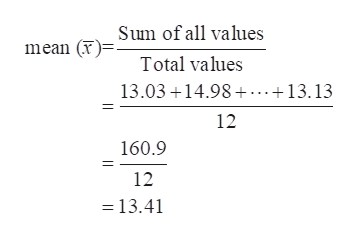# Eat your cereal: Boxes of cereal are labeled as containing 14 ounces. Following are the weights, in ounces, of a sample of 12 boxes. It is reasonable to assume that the population is approximately normal.13.0314.9813.1213.1313.1113.0313.1614.9813.0613.0513.1213.13The quality control manager is concerned that the mean weight is actually less than 14 ounces. Based on the confidence interval, is there a reason to be concerned? Explain.

Question
118 views

Eat your cereal: Boxes of cereal are labeled as containing 14 ounces. Following are the weights, in ounces, of a sample of 12 boxes. It is reasonable to assume that the population is approximately normal.

 13.03 14.98 13.12 13.13 13.11 13.03 13.16 14.98 13.06 13.05 13.12 13.13

The quality control manager is concerned that the mean weight is actually less than 14 ounces. Based on the confidence interval, is there a reason to be concerned? Explain.

check_circle

Step 1

According to the provided information, boxes of cereal are labeled as containing 14 ounces. In order to check the concern that mean weight is actually less than 14 ounces the required hypothesis can be constructed as:

Null hypothesis, there is no reason to be concerned that is the mean weight is actually equal to 14 ounces, H0:µ=14

Alternate hypothesis, there is a reason to be concerned that the mean weight is actually less than 14 ounces, H1:µ<14.

The sample size (n) = 12

The sample mean of the provided data can be obtained as:help_outlineImage TranscriptioncloseSum of all values mean ()= Total values 13.03 14.98 ...+13.13 12 160.9 12 13.41 fullscreen
Step 2

The sample standard deviation can be obtained as:

Step 3

The sample size is small (less than 30) and population standard deviation is unknown so the ...

### Want to see the full answer?

See Solution

#### Want to see this answer and more?

Solutions are written by subject experts who are available 24/7. Questions are typically answered within 1 hour.*

See Solution
*Response times may vary by subject and question.
Tagged in

### Hypothesis Testing